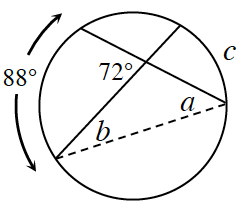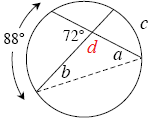### Home > CCG > Chapter 11 > Lesson 11.2.3 > Problem11-111

11-111.In part (c) of problem 11-110, you used the relationship between the segment lengths formed by intersecting chords to find a missing length. But how are the arc measures of two random intersecting chords related? Examine the diagram at right.

1. Solve for $a$$b$, and $c$ using what you know about inscribed angles and the sum of the angles of a triangle.

$88º = 2a$Look above at the image of the circle.
$72º + d = 180º$ because they are supplementary angles.

Use the Triangle Angle Sum Theorem to solve for $b$.

$c = (2)(b)$

1. Compare the result for $c$ with $88º$ and $72º$. Is there a relationship?

Since c is equal to $56º$, the measure of the vertical angles ($72º$) is the average of the measure of the two arcs that the vertical angles intercept.

$\frac{88 + 56}{2} = 72º$